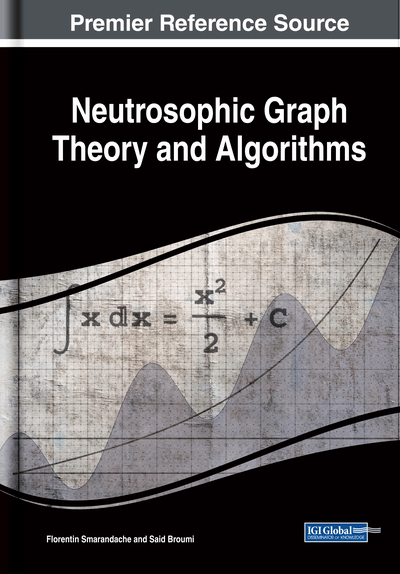# Distinguishable and Inverses of Neutrosophic Finite Automata

Kavikumar Jacob (University Tun Hussein Onn Malaysia, Malaysia), Nagarajan Deivanayagampillai (Hindustan Institute of Technology and Science, India), Lathamaheswari Malayalan (Hindustan Institute of Technology and Science, India), Gan Jian Yong (Universiti Tecknologi Malaysia, Malaysia) and Said Broumi (Faculty of Science Ben M'Sik, University Hassan II, Morocco)
DOI: 10.4018/978-1-7998-1313-2.ch011

## Abstract

This chapter focuses on neutrosophic finite automata with output function. Some new notions on neutrosophic finite automata are established and studied, such as distinguishable, rational states, semi-inverses, and inverses. Interestingly, every state in finite automata is said to be rational when its inputs are ultimately periodic sequence that yields an ultimately periodic sequence of outputs. This concludes that any given state is rational when its corresponding sequence of states is distinguishable. Furthermore, this study is to prove that the semi-inverses of two neutrosophic finite automata are indistinguishable. Finally, the result shows that any neutrosophic finite automata and its inverse are distinguished, and then their reverse relation is also distinguished.
Chapter Preview
Top

## Introduction

Automata are abstract machines for solving computational problems (Kafle & Gallagher 2017; Pan et.al. 2015). Fuzzy automata emerge from the inclusion of fuzzy logic into automata theory. Fuzzy finite automata are beneficial to model uncertainties which inherent in many applications (Doostfatemeh & Kremer 2005). Fuzzy finite automata with output offers further inclination in providing output compares to fuzzy finite automata. For each assigning input, the machine will generate output and its value is a function of the current state and the current input. Generally, in most applications and systems there is some kind of final output or decision. In the realm of deterministic and non-deterministic finite automata, there are no explicit outputs beyond the concept of acceptance attributed to each state. Hence, it is significant to assign output values to the states of fuzzy finite automata. In order to enhance the membership value of fuzzy automata into general algebraic strucutres, Li and Pedrycz (2005) study the concept of fuzzy automata based on lattice-ordered monoids. Jin et al. (2013) investigated the algebraic study of fuzzy automata based on po-monoids. Abolpour and Zahedi (2017) utlize the categorical concepts in the study of automata with membership values in different lattice structures.

In 1955, from the seminal paper by Mealy (1955) introduced Mealy automaton is a finite state machine with output, in which the state transitions are uniquely determined by the current state and input state. Further, Moore (1956) introduced a sequential machine based on output states, say, Moore automata. Mordeson and Nair (1996) have been introduced and studied a new case of automata that is so-called fuzzy Mealy machine. Verma and Tiwari (2017) recently introduced and studied the concepts of state distinguishability, input-distinguishability and output completeness of states of a crisp deterministic fuzzy automaton with output function. Huang et al. (2017) established the concepts of weak commutativity of a Mealy-type fuzzy finite state machine and studied its characterizations and properties. In 2018, Mealy-type and Moore-type weighted finite automata with output with respect to various semantics were studied by in (Ignjatovic et al. 2018).

The notions of neutrosophic sets was proposed by Smarandache (1999; 2006), generalizing the existing ordinary fuzzy sets, intuitionistic fuzzy sets and interval-valued fuzzy set in which each element of the universe has the degrees of truth, indeterminacy and falsity and the membership values are lies in ], the nonstandard unit interval (Rivieccio 2018) it is an extension from standard interval [0,1]. It has been shown that fuzzy sets provide a limited platform for computational complexity but neutrosophic sets are suitable for it. The neutrosophic set is an appropriate mechanism for interpreting real-life philosophical problems but not for scientific problems since it is difficult to consolidate. In neutrosophic sets, the degree of indeterminacy can be defined independently since it is quantified explicitly which led to different from intuitionistic fuzzy sets. As an application neutrosophic sets have been applied in many disciplines such as traffic control management (Nagarajan et al. 2019a; Nagarajan et al. 2019 b), graph theory (Tan et al. 2019; Jan et al. 2019; Broumi 2018) and decision-making (Abdel-Basset et al. 2019; Fahmi et al. 2018).

## Key Terms in this Chapter

Neutrosophic Output Function: Machine generates an output on every input.

Fuzzy Finite Automata: Fuzzy automata are used for handling the system uncertainty problem because classical automata cannot deal with system uncertainty.

Inverses: It considers the situation of one machine “undoing” the work of another.

Rational States: It is a state where each ultimately periodic sequence of inputs yields an ultimately periodic sequence of outputs.

Mealy Machine: It is a finite-state machine whose output values are determined both by its current state and the current inputs.

Lattice Ordered Monoid: It is generated by the natural numbers and satisfied the left cancellation law.

Indistinguishable States: It is one of method to reducing the states of a finite automaton is based on finding and combing indistinguishable states.

## Complete Chapter List

Search this Book:
Reset